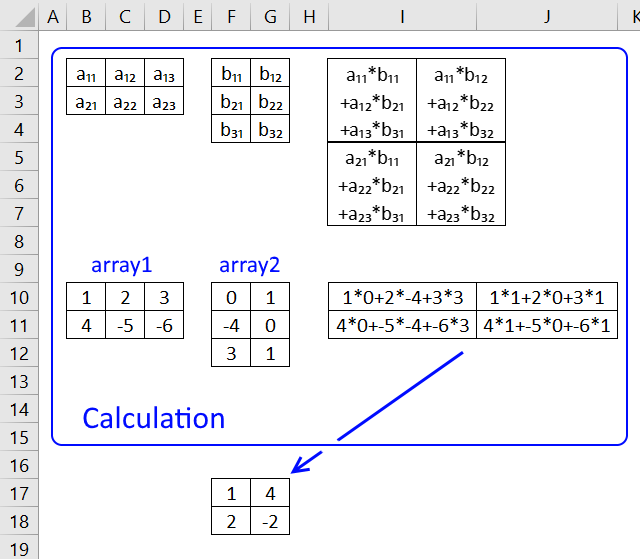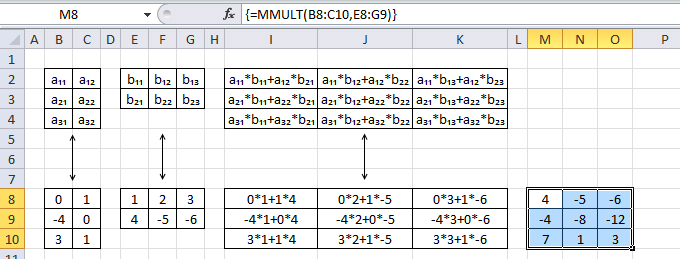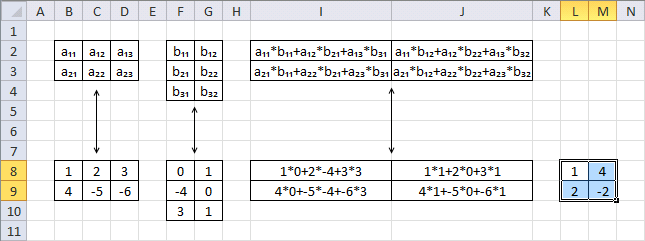Author: Oscar Cronquist Article last updated on April 19, 2018The MMULT function calculates the matrix product of two arrays, an array as the same number of rows as array1 and columns as array2.

### Excel Function Syntax

MMULT(array1, array2)

### Arguments

 array1 Required. The first array of numbers you want to multiply. array2 Required. The second array of numbers you want to multiply.

This function must be entered as an array formula.

The following examples demonstrate how the function works.

### Example 1

Array1 (B2:C3) has 2 rows and array2 (E2:F3) has 2 columns, the returning array has 2 rows and 2 columns.Array formula in cell range L8:M9:

=MMULT(B8:C9,E8:F9)

Cell L8: a₁₁*b₁₁+a₁₂*b₂₁ becomes 0*0+1*1 and returns 1

Cell L9: a₂₁*b₁₁+a₂₂*b₂₁ becomes 1*0+0*1 and returns 0

Cell M8: a₁₁*b₁₂+a₁₂*b₂₂ becomes 0*1+1*0 and returns 0

Cell M9: a₂₁*b₁₂+a₂₂*b₂₂ becomes 1*1+0*0 and returns 1

This post demonstrates how to refresh a scoreboard instantly using the MMULT function:

Dynamic scoreboard

Here comes another post about the MMULT function, today I made a dynamic scoreboard. There are five women competing and there […]

### Example 2

Array1 (B2:C4) has 3 rows and array2 (E2:G3) has 3 columns, the returning array has 3 rows and 3 columns.Array formula in cell range L8:M9:

=MMULT(B8:C10,E8:G9)

Cell M8: a₁₁*b₁₁+a₁₂*b₂₁ becomes 0*1+1*4 and returns 4

Cell M9: a₂₁*b₁₁+a₂₂*b₂₁ becomes -4*1+0*4 and returns -4

Cell M10: a₃₁*b₁₁+a₃₂*b₂₁ becomes 3*1+1*4 and returns 7

Cell N8: a₁₁*b₁₂+a₁₂*b₂₂ becomes 0*2+1*-5 and returns -5

Cell N9: a₂₁*b₁₂+a₂₂*b₂₂ becomes -4*2+0*-5 and returns -8

Cell N10: a₃₁*b₁₂+a₃₂*b₂₂ becomes 3*2+1*-5 and returns 1

Cell O8: a₁₁*b₁₃+a₁₂*b₂₃ becomes 0*3+1*-6 and returns -6

Cell O9: a₂₁*b₁₃+a₂₂*b₂₃ becomes -4*3+0*-6 and returns -12

Cell O10: a₃₁*b₁₃+a₃₂*b₂₃ becomes 3*3+1*-6 and returns 3

This article shows you how to find a set of numbers which adds up to a sum you specify:

Find numbers closest to sum

Excelxor is such a great website for inspiration, I am really impressed by this post Which numbers add up to […]

### Example 3

Array1 (B2:D3) has 2 rows and array2 (F2:G4) has 2 columns, the returning array has 2 rows and 2 columns.Array formula in cell range L8:M9:

=MMULT(B8:D9,F8:G10)

Cell L8: a₁₁*b₁₁+a₁₂*b₂₁+a₁₃*b₃₁ becomes 1*0+2*-4+3*3 and returns 1

Cell L9: a₂₁*b₁₁+a₂₂*b₂₁+a₂₃*b₃₁ becomes 4*0+-5*-4+-6*3 and returns 2

Cell M8: a₁₁*b₁₂+a₁₂*b₂₂+a₁₃*b₃₂ becomes 1*1+2*0+3*1 and returns 4

Cell M9: a₂₁*b₁₂+a₂₂*b₂₂+a₂₃*b₃₂ becomes 4*1+-5*0+-6*1 and returns -2

This article demonstrates how to search a data set with multiple strings:

Search each column for a string each and return multiple records – OR logic

RU asks: Can you please suggest if i want to find out the rows with fixed value in "First Name" […]